# Option pricing using the Black-Scholes model, without the formula

Every university student taking a module on finance has seen the Black-Scholes-Merton option pricing formula. It is long, ugly, and confusing. It doesn’t even give an intuition for pricing options. The derivation of it is so difficult that Scholes and Merton received a Nobel prize for it in 1997 (Black died in 1995). It relies on the Feynman-Kac theorem and risk-neutral measures, but I will not get into it

# Black-Scholes PDE

Pricing an option can be done using the Black-Scholes partial differential equation (BS PDE). The BS PDE can be derived by applying Ito’s Lemma to geometric Brownian motion and then setting the necessary conditions to satisfy the continuous-time delta hedging.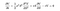Black-Scholes PDE.

# Numerical solutions to the PDE

As we are trying to solve the PDE numerically, we need to establish a few things before. Firstly, we will use it to value a European call option with strike price K. The method I will be using is called the finite difference method and it involves setting up a grid of points.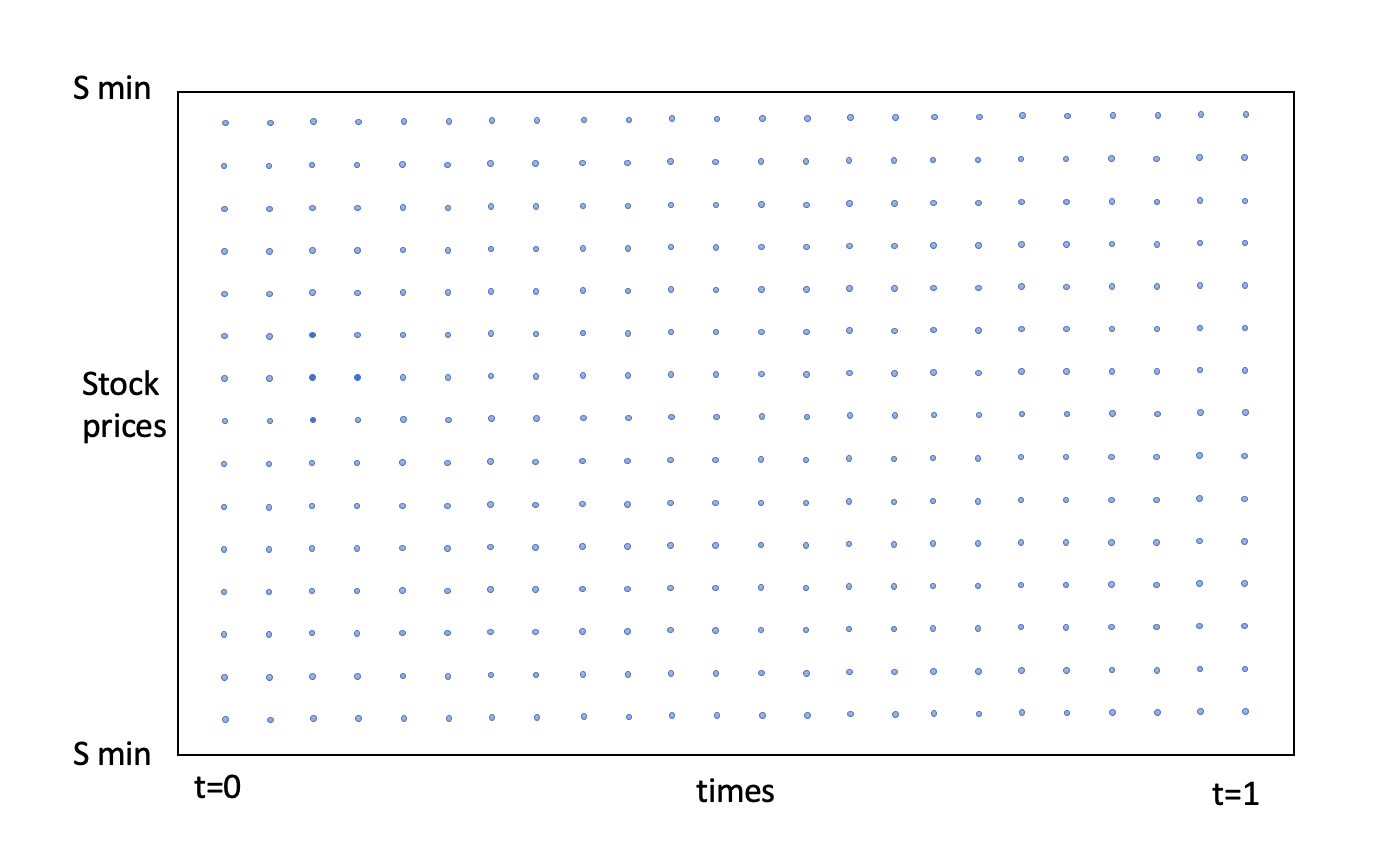Grid of points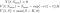Boundary conditions.Black-Scholes PDE.

# Estimating derivatives

With the discretized space, we can use central difference estimates for the derivatives of the option value (the delta and gamma from the greeks).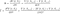Derivative approximations in the BS PDE.Black-Scholes PDE with central difference estimates.

## Simulation

Applying the Euler-Maruyama scheme to the discretized Black-Scholes PDE, we get: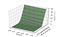Option price for K=50, r=0.02, sigma=0.2, N=M=50.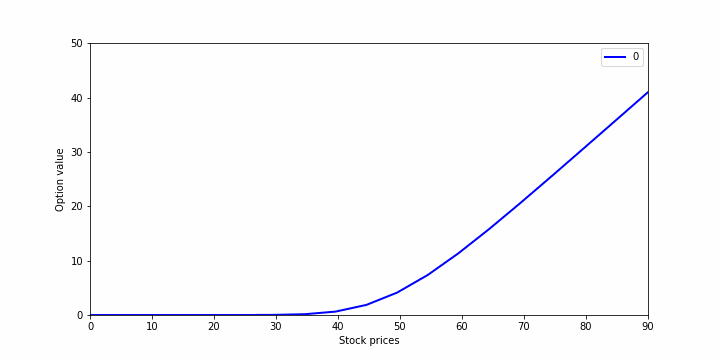Slice plot.

# Closing remarks

Pricing an option using the Black-Scholes PDE can be a very good intuition building example, but sadly it cannot really be used in practice. Mainly because it is slow to use and we have the formula to use. My above method can be made more robust by tuning the Crank-Nicholson method to simulate, which makes the process less sensitive.

Interested in applied maths, quantitative finance, and game theory. https://www.linkedin.com/in/daniel-reti-22319b168/

## More from Daniel Reti

Interested in applied maths, quantitative finance, and game theory. https://www.linkedin.com/in/daniel-reti-22319b168/